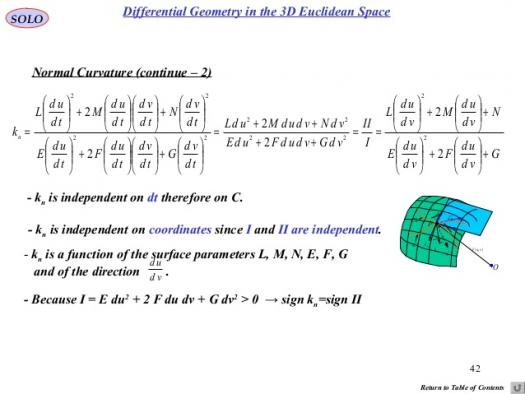# What Is Percent Problems?

Approved & Edited by ProProfs Editorial Team
The editorial team at ProProfs Quizzes consists of a select group of subject experts, trivia writers, and quiz masters who have authored over 10,000 quizzes taken by more than 100 million users. This team includes our in-house seasoned quiz moderators and subject matter experts. Our editorial experts, spread across the world, are rigorously trained using our comprehensive guidelines to ensure that you receive the highest quality quizzes.
| By Livyn
L
Livyn
Community Contributor
Quizzes Created: 347 | Total Attempts: 144,443
Questions: 10 | Attempts: 178SettingsTo solve percent problems, you can use the equation,Percent. Base = Amount, and solve for the unknown numbers. Or, you can set up the proportion, Percent = , where the percent is a ratio of a number to 100. You can then use cross multiplication to solve the proportion.

• 1.

### Percentage is a topic in ....?

• A.

Maths

• B.

English Language

• C.

Literature

• D.

Music

A. Maths
• 2.

### In mathematics, a percentage is a number or .... expressed as a fraction.

• A.

Decimals

• B.

Ratio

• C.

Algebra

• D.

Indice

B. Ratio
Explanation
A percentage is a way of expressing a fraction or a part of a whole in terms of a ratio. It represents a proportion or comparison between two quantities.

Rate this question:

• 3.

### In mathematics, a percentage is a number or ratio expressed as a fraction of ....?

• A.

100

• B.

1000

• C.

10

• D.

1

A. 100
Explanation
A percentage is a number or ratio expressed as a fraction of 100. It is a way to express a part of a whole in terms of hundredths. For example, if you have 50 out of 100, it can be written as 50%. Therefore, the correct answer is 100.

Rate this question:

• 4.

### It is often denoted using the percent sign, ....?

• A.

?

• B.

/

• C.

%

• D.

@

C. %
Explanation
The percent sign is often used to denote percentages. Percentages are a way of expressing a proportion or a fraction out of 100. For example, 50% means 50 out of 100, or half. The percent sign is widely recognized and understood as a symbol for percentages in mathematics, finance, and other fields.

Rate this question:

• 5.

### Sometimes the abbreviation "...." is also used.

• A.

Pct

• B.

Prcnt

• C.

Pcnt

• D.

Percnt

A. Pct
Explanation
The abbreviation "pct" is sometimes used as an alternative to "percent".

Rate this question:

• 6.

### One can express a number as a fraction of 1,000 using the term "....."

• A.

Per kilo

• B.

Per mille

• C.

Per meter

• D.

Per hundred

B. Per mille
Explanation
The term "per mille" is used to express a number as a fraction of 1,000. It is similar to "percent" but with a base of 1,000 instead of 100. For example, if a number is expressed as "5 per mille," it means that it is equal to 5/1000 or 0.005.

Rate this question:

• 7.

### In ....., long before the existence of the decimal system, computations were often made in fractions which were multiples of ​1⁄100.

• A.

Ancient Britain

• B.

Ancient Greece

• C.

Ancient Rome

• D.

Ancient Nigeria

C. Ancient Rome
Explanation
In Ancient Rome, long before the existence of the decimal system, computations were often made in fractions which were multiples of ​1⁄100. This suggests that the Romans had a sophisticated understanding of fractions and used them in their calculations. The use of fractions in computations was likely a common practice in Ancient Rome, indicating their advanced mathematical knowledge and skills.

Rate this question:

• 8.

### Who levied a tax of ​1⁄100 on goods sold at auction known as centesima rerum venalium?

• A.

Brutus

• B.

Caesar

• C.

Augustus

• D.

Pythagoras

C. Augustus
Explanation
Augustus levied a tax of ​1/100 on goods sold at auction known as centesima rerum venalium. This tax was implemented during his reign as the first Roman emperor. Augustus introduced various reforms to stabilize and strengthen the Roman economy, and the centesima rerum venalium tax was one of his measures to generate revenue for the state.

Rate this question:

• 9.

### The term "per cent" is derived from .....?

• A.

Latin

• B.

Greek

• C.

English

• D.

French

A. Latin
Explanation
The term "per cent" is derived from Latin. The word "per centum" in Latin means "by the hundred." This term is used to express a proportion or a fraction out of 100. It has been widely adopted in various languages, including English, to represent percentages.

Rate this question:

• 10.

### By what century was it standard to quote interest rates in hundredths?

• A.

11th Century

• B.

15th Century

• C.

17th Century

• D.

20th Century

C. 17th Century
Explanation
In the 17th century, it became standard to quote interest rates in hundredths. This means that instead of using fractions or other forms of representation, interest rates were expressed as a percentage out of 100. This standardized approach made it easier for people to compare and understand interest rates, leading to more efficient financial transactions and calculations.

Rate this question:

Related TopicsBack to top